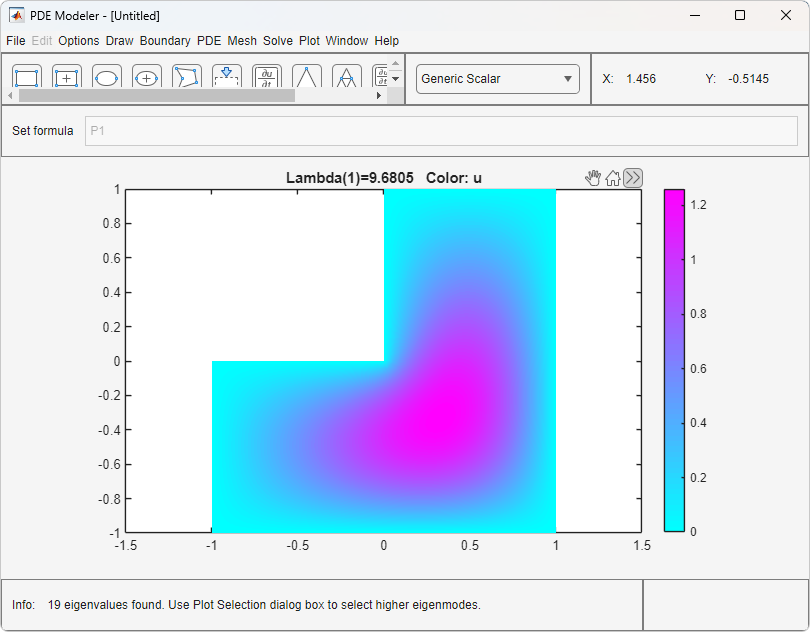Documentation

# PDE Modeler

Create complex 2-D geometries by drawing, overlapping, and rotating basic shapes

## Description

The PDE Modeler app provides an interactive interface for solving 2-D geometry problems. Using the app, you can create complex geometries by drawing, overlapping, and rotating basic shapes, such as circles, polygons and so on. The app also includes preset modes for applications, such as electrostatics, magnetostatics, heat transfer, and so on.

When solving a PDE problem in the app, follow these steps:

1. Create a 2-D geometry.

2. Specify boundary conditions.

3. Specify equation coefficients.

4. Generate a mesh.

5. Specify parameters for solving a PDE. The set of parameters depends on the type of PDE. For parabolic and hyperbolic PDEs, these parameters include initial conditions.

6. Solve the problem.

7. Specify plotting parameters and plot the results.

You can choose to export data to the MATLAB® workspace from any step in the app and continue your work outside the app.

### Note

The app does not support 3-D geometry problems and systems of more than two PDEs.## Open the PDE Modeler App

• MATLAB Toolstrip: On the Apps tab, under Math, Statistics and Optimization, click the app icon.

• MATLAB command prompt: Enter `pdeModeler`.

## Programmatic Use

expand all

`pdeModeler` opens the PDE Modeler app or brings focus to the app if it is already open.

`pdecirc(xc,yc,r)` opens the PDE Modeler app and draws a circle with center in `(xc,yc)` and radius `r`.

`pdeellip(xc,yc,a,b,phi)` opens the PDE Modeler app and draws an ellipse with center in `(xc,yc)` and semiaxes `a` and `b`. The rotation of the ellipse (in radians) is `phi`.

`pdepoly(x,y)` opens the PDE Modeler app and draws a polygon with corner coordinates defined by `x` and `y`.

`pderect([xmin xmax ymin ymax])` opens the PDE Modeler app and draws a rectangle with corner coordinates defined by ```[xmin xmax ymin ymax]```.